# interpolateCurrentDensity

Interpolate current density in DC conduction result at arbitrary spatial locations

Since R2022b

## Syntax

``Jintrp = interpolateCurrentDensity(results,xq,yq)``
``Jintrp = interpolateCurrentDensity(results,xq,yq,zq)``
``Jintrp = interpolateCurrentDensity(results,querypoints)``

## Description

example

````Jintrp = interpolateCurrentDensity(results,xq,yq)` returns the interpolated current density values at the 2-D points specified in `xq` and `yq`.```

example

````Jintrp = interpolateCurrentDensity(results,xq,yq,zq)` uses 3-D points specified in `xq`, `yq`, and `zq`.```

example

````Jintrp = interpolateCurrentDensity(results,querypoints)` returns the interpolated current density values at the points specified in `querypoints`.```

## Examples

collapse all

Create an electromagnetic model for DC conduction analysis.

`model = createpde("electromagnetic","conduction");`

Import and plot the geometry representing a plate with a hole.

```importGeometry(model,"PlateHolePlanar.stl"); figure pdegplot(model,"EdgeLabels","on");```Specify the conductivity of the material.

`electromagneticProperties(model,"Conductivity",6e4);`

Apply the voltage boundary conditions on the top and bottom edges of the plate.

```electromagneticBC(model,"Voltage",100,"Edge",3); electromagneticBC(model,"Voltage",200,"Edge",2);```

Specify the surface current density on the edge representing the hole.

`electromagneticBC(model,"SurfaceCurrentDensity",200000,"Edge",5);`

Generate the mesh.

`generateMesh(model);`

Solve the model.

`R = solve(model);`

Plot the electric potential and current density.

```figure pdeplot(model,"XYData",R.ElectricPotential,"ColorMap","jet", ... "FlowData",[R.CurrentDensity.Jx R.CurrentDensity.Jy]) axis equal```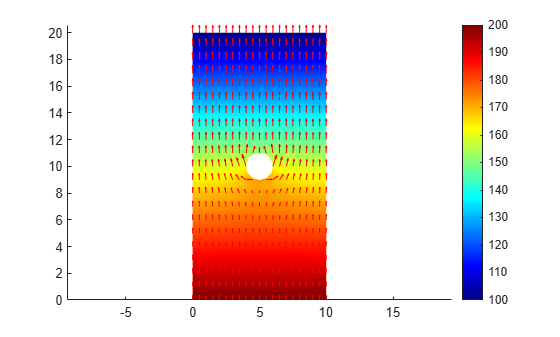Interpolate the resulting current density to a grid covering the central portion of the geometry.

```[X,Y] = meshgrid(2:0.25:8,8:0.25:12); Jintrp = interpolateCurrentDensity(R,X,Y)```
```Jintrp = FEStruct with properties: Jx: [425x1 double] Jy: [425x1 double] ```

Reshape `Jintrp.Jx` and `Jintrp.Jy`, and plot the resulting current density.

```JintrpX = reshape(Jintrp.Jx,size(X)); JintrpY = reshape(Jintrp.Jy,size(Y)); quiver(X,Y,JintrpX,JintrpY,"Color","red") axis equal```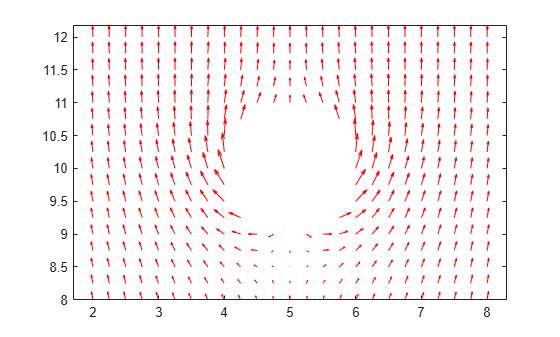Alternatively, you can specify the grid by using a matrix of query points.

```querypoints = [X(:),Y(:)]'; Jintrp = interpolateCurrentDensity(R,querypoints);```

Create an electromagnetic model for DC conduction analysis.

`model = createpde("electromagnetic","conduction");`

Import and plot the geometry representing a 10-by-10-by-1 solid plate.

```g = importGeometry(model,"Plate10x10x1.stl"); pdegplot(model,"FaceLabels","on","FaceAlpha",0.3)```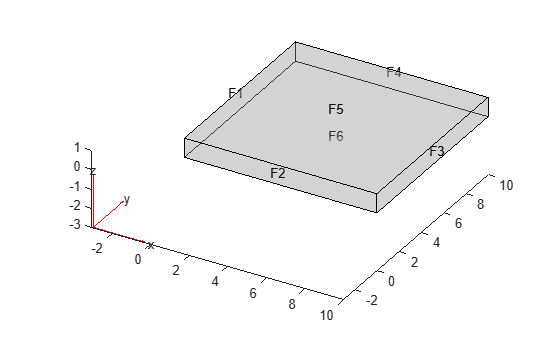Specify the conductivity of the material.

`electromagneticProperties(model,"Conductivity",6e4);`

Apply the voltage boundary conditions on the two faces of the plate.

`electromagneticBC(model,"Voltage",0,"Face",[1 3]);`

Specify the surface current density on the top of the plate.

`electromagneticBC(model,"SurfaceCurrentDensity",100,"Face",5);`

Generate the mesh.

`generateMesh(model);`

Solve the model.

`R = solve(model);`

Plot the electric potential.

```figure pdeplot3D(model,"ColorMapData",R.ElectricPotential)```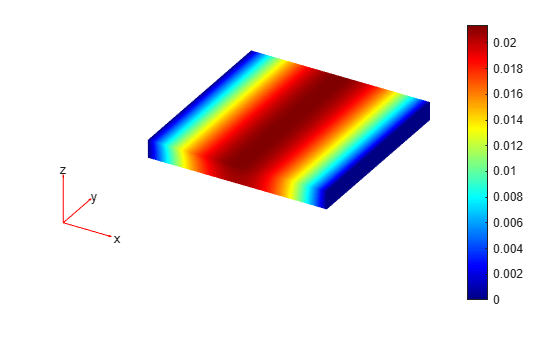Plot the current density.

```figure pdeplot3D(model,"FlowData",[R.CurrentDensity.Jx, ... R.CurrentDensity.Jy, ... R.CurrentDensity.Jz])```Interpolate the resulting current density to a coarser grid.

```[X,Y,Z] = meshgrid(0:10,0:10,0:0.5:1); Jintrp = interpolateCurrentDensity(R,X,Y,Z)```
```Jintrp = FEStruct with properties: Jx: [363x1 double] Jy: [363x1 double] Jz: [363x1 double] ```

Reshape `Jintrp.Jx`, `Jintrp.Jy`, and `Jintrp.Jz`.

```JintrpX = reshape(Jintrp.Jx,size(X)); JintrpY = reshape(Jintrp.Jy,size(Y)); JintrpZ = reshape(Jintrp.Jz,size(Z));```

Plot the resulting current density.

```figure quiver3(X,Y,Z,JintrpX,JintrpY,JintrpZ,"Color","red")```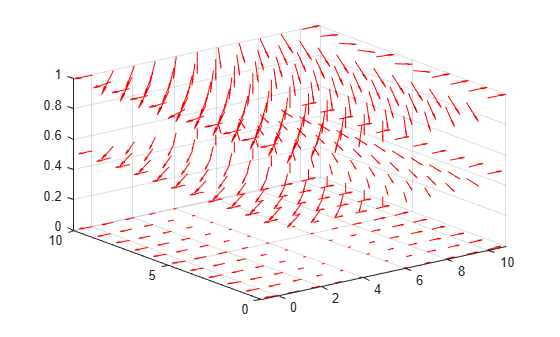## Input Arguments

collapse all

Solution of a DC conduction problem, specified as a `ConductionResults` object. Create `results` using the `solve` function.

Example: `results = solve(emagmodel)`

x-coordinate query points, specified as a real array. `interpolateCurrentDensity` evaluates the current density at the 2-D coordinate points `[xq(i) yq(i)]` or at the 3-D coordinate points `[xq(i) yq(i) zq(i)]` for every `i`. Because of this, `xq`, `yq`, and (if present) `zq` must have the same number of entries.

`interpolateCurrentDensity` converts the query points to column vectors `xq(:)`, `yq(:)`, and (if present) `zq(:)`. It returns current density values as a column vector of the same size. To ensure that the dimensions of the returned solution are consistent with the dimensions of the original query points, use `reshape`. For example, use `Jintrp = reshape(Jintrp,size(xq))`.

Example: `xq = [0.5 0.5 0.75 0.75]`

Data Types: `double`

y-coordinate query points, specified as a real array. `interpolateCurrentDensity` evaluates the current density at the 2-D coordinate points `[xq(i) yq(i)]` or at the 3-D coordinate points `[xq(i) yq(i) zq(i)]` for every `i`. Because of this, `xq`, `yq`, and (if present) `zq` must have the same number of entries.

`interpolateCurrentDensity` converts the query points to column vectors `xq(:)`, `yq(:)`, and (if present) `zq(:)`. It returns current density values as a column vector of the same size. To ensure that the dimensions of the returned solution are consistent with the dimensions of the original query points, use `reshape`. For example, use `Jintrp = reshape(Jintrp,size(yq))`.

Example: `yq = [1 2 0 0.5]`

Data Types: `double`

z-coordinate query points, specified as a real array. `interpolateCurrentDensity` evaluates the current density at the 3-D coordinate points `[xq(i) yq(i) zq(i)]`. Therefore, `xq`, `yq`, and `zq` must have the same number of entries.

`interpolateCurrentDensity` converts the query points to column vectors `xq(:)`, `yq(:)`, and `zq(:)`. It returns current density values as a column vector of the same size. To ensure that the dimensions of the returned solution are consistent with the dimensions of the original query points, use `reshape`. For example, use `Jintrp = reshape(Jintrp,size(zq))`.

Example: `zq = [1 1 0 1.5]`

Data Types: `double`

Query points, specified as a real matrix with either two rows for 2-D geometry or three rows for 3-D geometry. `interpolateCurrentDensity` evaluates the current density at the coordinate points `querypoints(:,i)` for every `i`, so each column of `querypoints` contains exactly one 2-D or 3-D query point.

Example: For a 2-D geometry, ```querypoints = [0.5 0.5 0.75 0.75; 1 2 0 0.5]```

Data Types: `double`

## Output Arguments

collapse all

Current density at query points, returned as an `FEStruct` object with the properties representing the spatial components of the current density at the query points. For query points that are outside the geometry, `Jintrp.Jx(i)`, `Jintrp.Jy(i)`, and `Jintrp.Jz(i)` are `NaN`. Properties of an `FEStruct` object are read-only.

## Version History

Introduced in R2022b